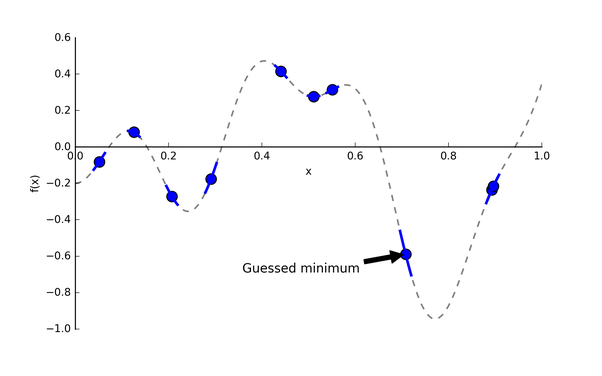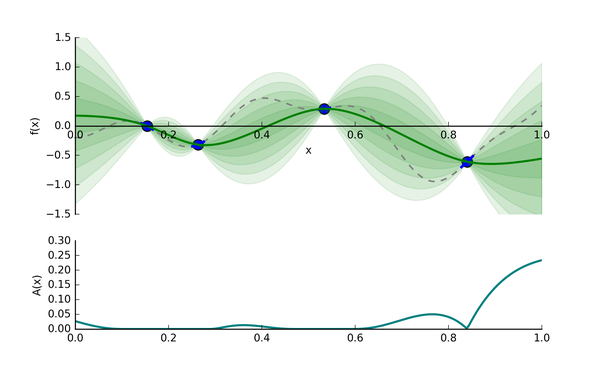﻿ 详解Python中4种超参自动优化算法的实现_python_脚本之家
python# 详解Python中4种超参自动优化算法的实现

## 一、网格搜索(Grid Search)

```from sklearn import svm, datasets
from sklearn.model_selection import GridSearchCV
import pandas as pd

# 导入数据
# 定义超参搜索空间
parameters = {'kernel':('linear', 'rbf'), 'C':[1, 10]}
# 初始化模型
svc = svm.SVC()
# 网格搜索
clf = GridSearchCV(estimator = svc,
param_grid = parameters,
scoring = 'accuracy',
n_jobs = -1,
cv = 5)
clf.fit(iris.data, iris.target)

param_grid={'C': [1, 10], 'kernel': ('linear', 'rbf')},
scoring='accuracy')

# 打印结果
print('详细结果:\n', pd.DataFrame.from_dict(clf.cv_results_))
print('最佳分类器:\n', clf.best_estimator_)
print('最佳分数:\n', clf.best_score_)
print('最佳参数:\n', clf.best_params_).

mean_fit_time  std_fit_time  mean_score_time  std_score_time param_C  ... split3_test_score split4_test_score  mean_test_score  std_test_score  rank_test_score
0       0.000788      0.000394         0.000194        0.000389       1  ...          0.966667               1.0         0.980000        0.016330                1
1       0.000804      0.000402         0.000199        0.000399       1  ...          0.933333               1.0         0.966667        0.021082                4
2       0.000593      0.000484         0.000593        0.000484      10  ...          0.966667               1.0         0.973333        0.038873                3
3       0.000593      0.000484         0.000399        0.000489      10  ...          0.966667               1.0         0.980000        0.016330                1
[4 rows x 15 columns]

SVC(C=1, kernel='linear')

0.9800000000000001

{'C': 1, 'kernel': 'linear'}
```

sklearn.model_selection.GridSearchCV的重要参数说明:

• estimator: scikit-learn模型。
• param_grid: 超参搜索空间，即超参数字典。
• scoring: 在交叉验证中使用的评估策略。
• n_jobs: 并行任务数，-1为使用所有CPU。
• cv: 决定采用几折交叉验证。

## 二、随机搜索(Randomized Search)```from sklearn import svm, datasets
from sklearn.model_selection import RandomizedSearchCV
import pandas as pd
from scipy.stats import uniform

# 导入数据
# 定义超参搜索空间
distributions = {'kernel':['linear', 'rbf'], 'C':uniform(loc=1, scale=9)}
# 初始化模型
svc = svm.SVC()
# 网格搜索
clf = RandomizedSearchCV(estimator = svc,
param_distributions = distributions,
n_iter = 4,
scoring = 'accuracy',
cv = 5,
n_jobs = -1,
random_state = 2021)
clf.fit(iris.data, iris.target)

param_distributions={'C': <scipy.stats._distn_infrastructure.rv_frozen object at 0x000001F372F9A190>,
'kernel': ['linear', 'rbf']},
random_state=2021, scoring='accuracy')

# 打印结果
print('详细结果:\n', pd.DataFrame.from_dict(clf.cv_results_))
print('最佳分类器:\n', clf.best_estimator_)
print('最佳分数:\n', clf.best_score_)
print('最佳参数:\n', clf.best_params_)

mean_fit_time  std_fit_time  mean_score_time  std_score_time  param_C  ... split3_test_score split4_test_score  mean_test_score  std_test_score  rank_test_score
0       0.000598      0.000489         0.000200        0.000400   6.4538  ...          0.966667               1.0         0.986667        0.016330                1
1       0.000997      0.000002         0.000000        0.000000  4.99782  ...          0.966667               1.0         0.980000        0.026667                3
2       0.000798      0.000399         0.000399        0.000488  3.81406  ...          0.966667               1.0         0.980000        0.016330                3
3       0.000598      0.000488         0.000200        0.000399  5.36286  ...          0.966667               1.0         0.986667        0.016330                1
[4 rows x 15 columns]

SVC(C=6.453804509266643)

0.9866666666666667

{'C': 6.453804509266643, 'kernel': 'rbf'}
```

## 三、贝叶斯优化(Bayesian Optimization)(1) 已开发区域: 在绿色实线上最低的超参点。因为很可能它附近存在全局最优点。

(2) 未探索区域: 绿色实线上还未被探索的区域。比如图4，相比于0.15-0.25区间，0.65-0.75区间更具有探索价值(即该区间Uncertainty更大)。探索该区域有利于减少我们猜测的方差。

(1) 减少均值函数 ，提高EI。

(2) 增加方差 ，提高EI。SMBO是简洁版的贝叶斯优化，伪代码如图6所示，具体如下：

(1) 准备输入: expensive blackbox function ，超参搜索空间 ，采样数据集 (超参组合 ，对应 输出值)，采集函数 和用数据集 拟合的代理模型M。

(2) 基于 和 ，采样得到数据集 。

(3) 循环选 次参数：

```from sklearn import svm, datasets
from sklearn.model_selection import cross_val_score
from hyperopt import hp, fmin, tpe, space_eval
import pandas as pd

# 导入数据

# step1: 定义目标函数
def objective(params):
# 初始化模型并交叉验证
svc = svm.SVC(**params)
cv_scores = cross_val_score(svc, iris.data, iris.target, cv=5)
# 返回loss = 1 - accuracy (loss必须被最小化)
loss = 1 - cv_scores.mean()
return loss

# step2: 定义超参搜索空间
space = {'kernel':hp.choice('kernel', ['linear', 'rbf']),
'C':hp.uniform('C', 1, 10)}

# step3: 在给定超参搜索空间下，最小化目标函数
best = fmin(objective, space, algo=tpe.suggest, max_evals=100)

# step4: 打印结果
print(best)

```

## 四、Hyperband## 总结

As a general rule of thumb, any time you want to optimize tuning hyperparameters, think Grid Search and Randomized Search! 

Hyperparameter Optimization for Machine Learning Models - Nagesh Singh Chauhan